### I) Brief introduction

The gradient expansion (GE) of the exchange-correlation (xc) energy of density functional theory includes the inhomogeneity corrections to the xc-energy of the homogeneous electron gas in a systematic fashion. Its basic ingredients are the gradients of the ground state (spin-)density n(r). The lowest order contribution to the GE, depending on [grad(n)]2, is rigorously determined by the long wavelength limit of the linear response function of the homogeneous electron gas. The first principles evaluation of higher order gradient terms requires knowledge of higher order contributions to the long wavelength expansion of the linear response functions and of nonlinear response.

The convergence of the GE (or the GE-based functionals) and thus its applicability depend on the actual size of the gradients. In practice, of course, only the lowest gradients are of interest. The appropriate dimensionless form of these gradients is obtained by analysis of the long-wavelength expansions of the response functions. For the first gradient one finds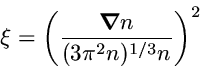while the second gradient enters the GE in the form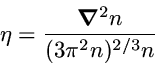In terms of these quantities the exchange functional has a particularly simple form,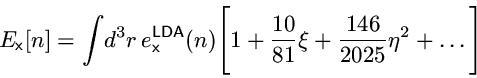The convergence of the GE thus requires that the product of the characteristic gradients with the corresponding prefactors is smaller than 1. As the prefactors turn out to be smaller than about 0.1 the gradients should be of the order of (or smaller than) 1. Below these quantities are shown for a number of systems, ranging from atoms to solids. The densities used for these plots have been obtained by selfconsistent calculations with the exact exchange. However, the characteristic gradients are rather insensitive to the particular density utilized.

The units of the plots are as follows: All length scales are given in Bohr. The characteristic gradients themselves are dimensionless.

### II) Results for atoms

#### II.1) Oxygen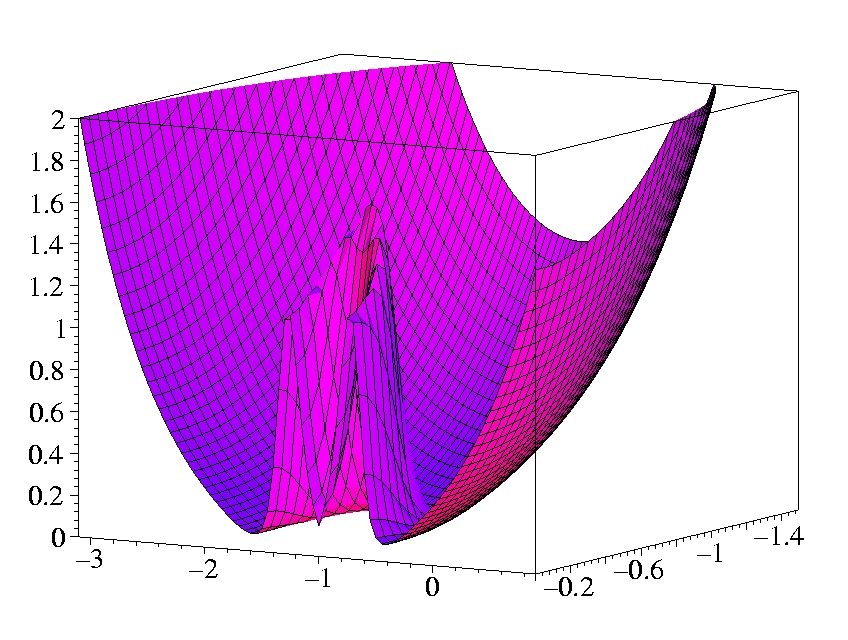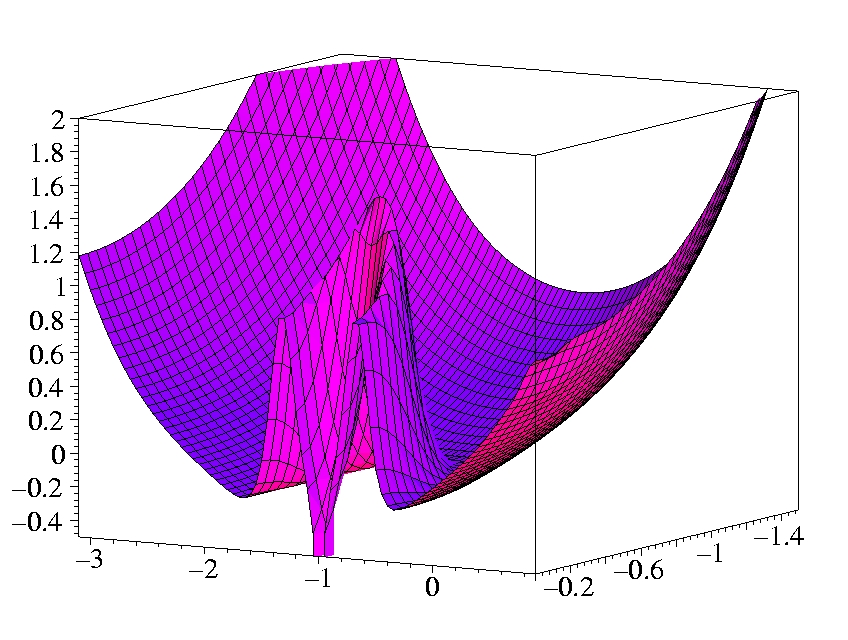• The plots show the gradients in the x-z-plane. The atom is sitting on the x-axis at z = -1 Bohr.
• The shell structure is obvious in both gradients.
• The Laplacian of the density diverges at the position of the nucleus (cusp condition).
• Both gradients diverge for exponentially decaying density.

see
E. Engel and S. H. Vosko, Phys. Rev. B 47, 13164 (1993).

### III) Results for molecules

#### III.1) H2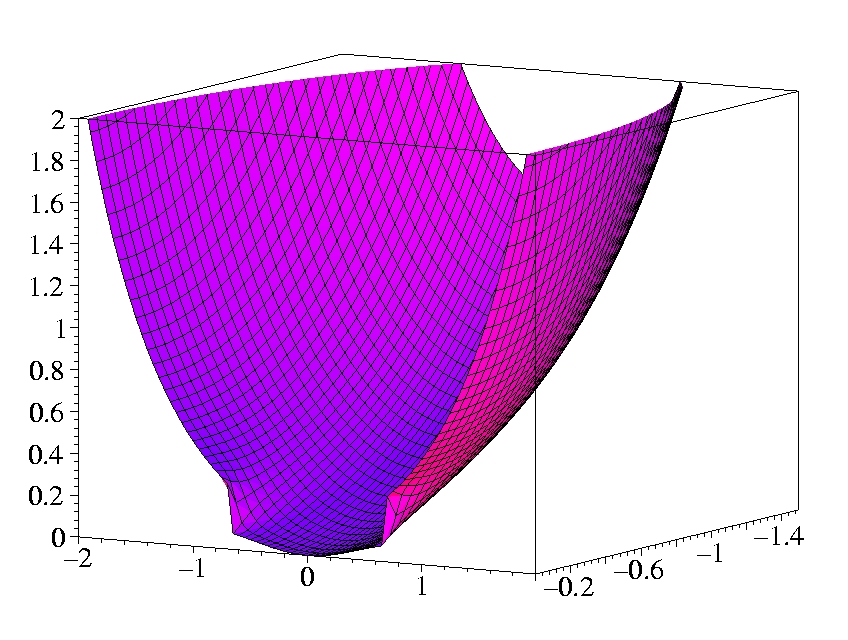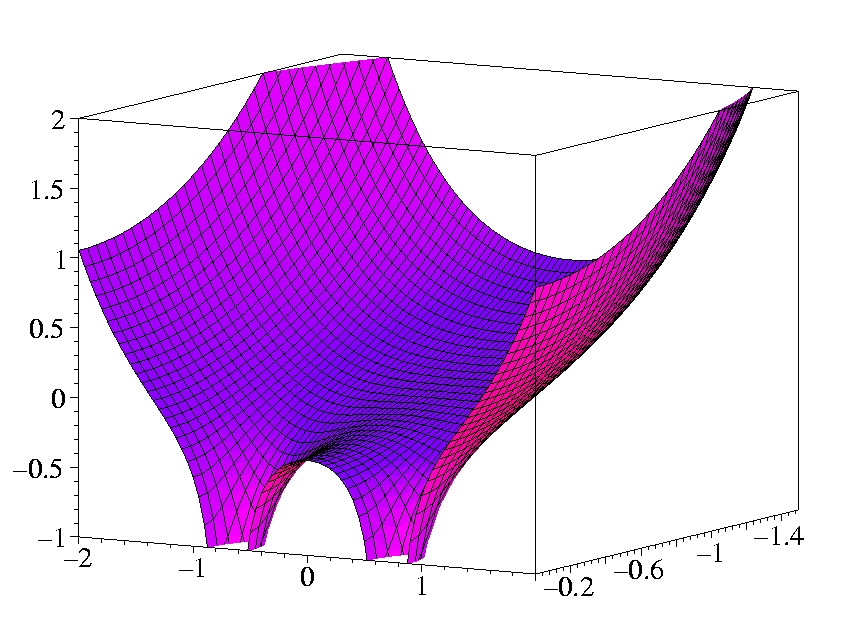#### III.2) N2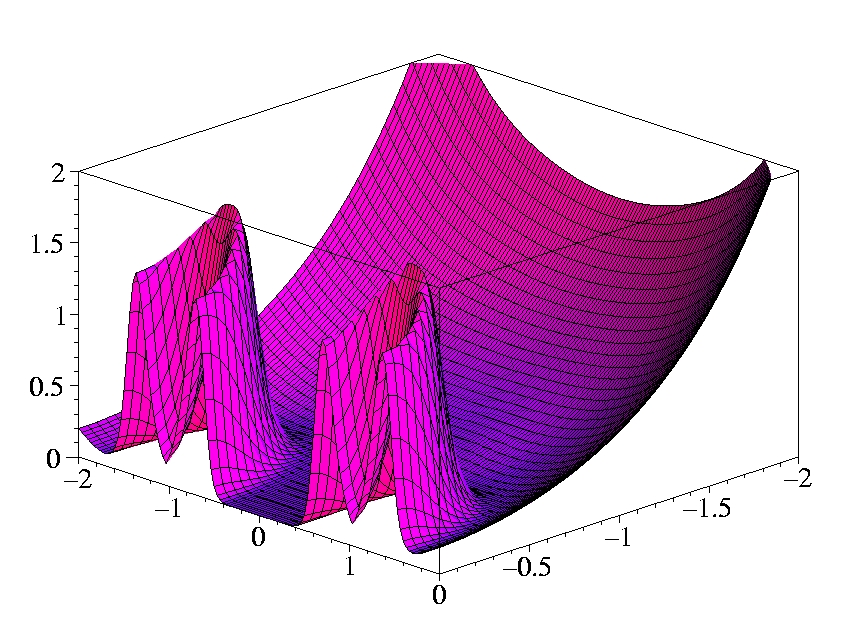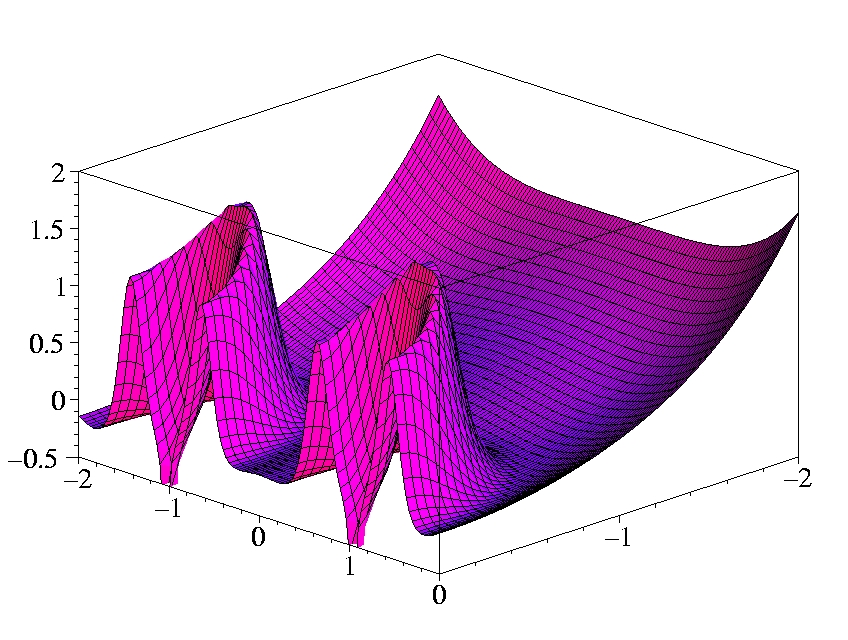### IV) Results for solids

#### IV.1) Silicon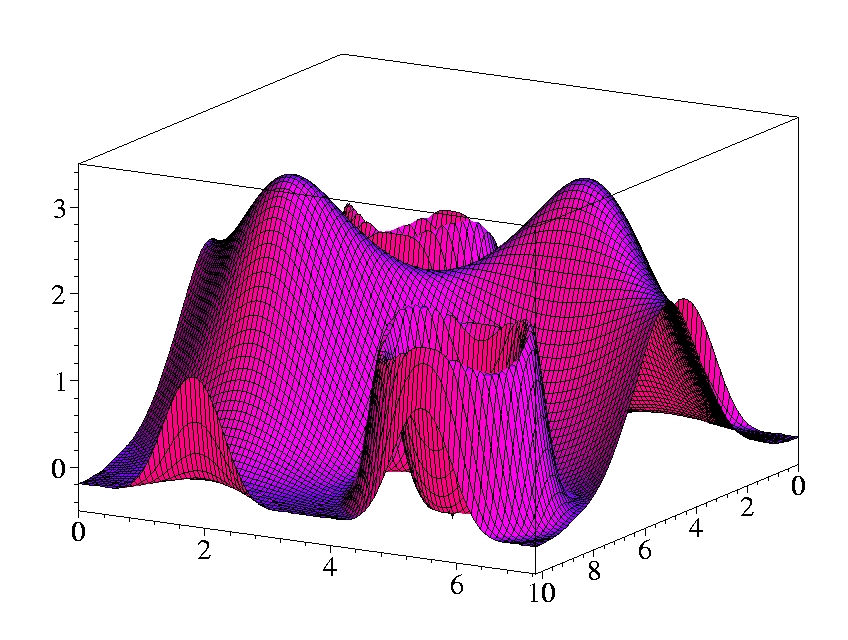#### IV.2) Gold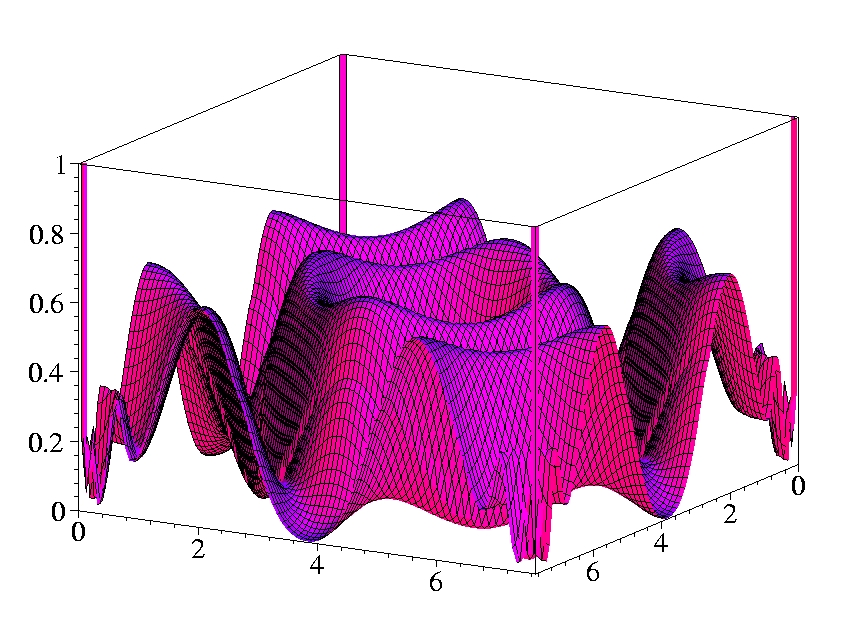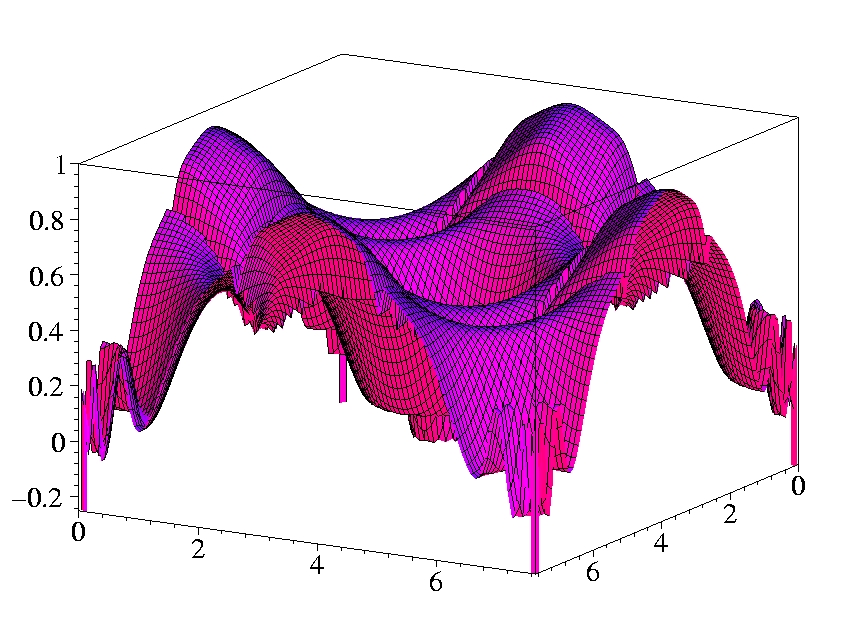Home Top